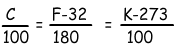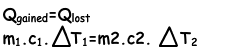## Heat Temperature and Expansion Cheatsheet

Heat Temperature and Expansion Cheat Sheet

Temperature

In an object all particles have kinetic energies because of their random motions. Temperature is the quantity which is directly proportional to the average kinetic energy of the atoms of matter. We measure temperature of matters with a device called thermometer. There are three types of thermometer, Celsius Thermometer, Fahrenheit Thermometer and Kelvin Thermometer.

We can convert the measurements of Celsius to Kelvin or Fahrenheit to Kelvin, Celsius by using following equations;Heat

Heat is a form of energy that flows from hotter substance to colder one. We mean by hotter and colder substance, substance having high temperature and low temperature with respect to a reference matter. There must be a difference in temperatures of the substance to have heat or energy transfer.

Specific Heat Capacity

Each matter has its own characteristics to absorb heat. We call this concept as specific heat capacity of the matters. It is the distinguishing property of matters. We show it with the letter “c” and give the definition of it as, heat required to increase temperature of unit mass 1 Cº. On the contrary, heat capacity of the system is defined as “heat required increasing the temperature of whole substance” and we show it with “C”.

With the help of specific heat capacity and mass of the matter we can find the relation between heat and change in the temperature in the given formula below.

Q=m.c.∆T

Where; Q is heat, m is mass, c is specific heat capacity and ∆T is the change in the temperature.

Heat Transfer

In previous section we have talked about heat. We said that, heat flows from the warmer objects to cooler ones. Heat transfer occurs in three ways, convection, conduction and radiation.

Calculations with Heat Transfer

If two objects having different temperatures are in contact, heat transfer starts between them. The amount of heat given is equal to the amount of heat taken. Object one has mass m1, temperature t1 and specific heat capacity c1, object two has mass m2, temperature t2 and specific heat capacity c2.Change of Phase/State

Matters can be in four states like solid, liquid, gas and plasma. Distance between the molecules or atoms of the matter shows its state or phase. Temperature and pressure are the only factors that affect the phases of matter.

Melting and Freezing

If solid matters gain enough heat they change state solid to liquid. We call this process melting and inverse of this process is called freezing.

Evaporation

Evaporation is the change of phase from liquid to gas. Evaporation occurs only at the surface of the water and at every temperature.

Sublimation

Sublimation is the change of state from solid to gas.

Thermal Expansion and Contraction

Most of the matters, without some exceptions, expand with the increasing temperature. When you give heat to matters; speed of its particles increase and distance between them also increase which results in the increase of the volumes of matters. Inverse of the expansion is called contraction, generally when matters lost heat and their temperatures decrease they contract.

We get following formula from the explanations given above;

∆V=V0.α. ∆T

Where; V is the change in the volume, α is the coefficient of thermal expansion and ∆T is the change in the temperature of the matter.

α= Coefficient of thermal expansion is equal to the change in the volume of a unit of mass under 10C change in temperature.

Linear Expansion: When you give heat to rod, its length increases.

∆L=L0.α. ∆T

Area Expansion: When plate is heated, it expands in two dimensions X and Y. We find the area expansion with the given formula;

∆S=S0.2α. ∆T

Volume Expansion: If the objects expand in volume with the gained heat, we call this situation "volume expansion" and find it with the following formula;

∆V=V0.3α. ∆T

Heat Temperature and Thermal Expansion Exams and Solutions

Author: